##### Actions

(diff) ← Older revision | Latest revision (diff) | Newer revision → (diff)

Letbe a CW-spectrum (see Spectrum of spaces) and considerwhereis another CW-spectrum,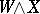is the smash product ofand(see [a2], Sect. III.4),is the sphere spectrum, and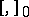denotes stable homotopy classes of mappings of spectra. Withfixed, this is a contravariant functor ofwhich satisfies the axioms of E.H. Brown (see [a1]) and which is hence representable by a spectrum, the Spanier–Whitehead dual of.is a contravariant functor with many duality properties. E.g.,

i)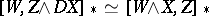;

ii)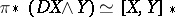;

iii)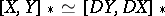;

iv)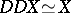;

v) for a (generalized) homology theorythere is a natural isomorphism between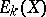and.

In many ways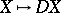is similar to the linear duality functorfor finite-dimensional vector spaces over a field.

For, the-dimensional sphere, the classical Alexander duality theorem says thatis isomorphic to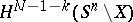, and this forms the basic intuitive geometric idea behind Spanier–Whitehead duality.

For more details, see [a2], Sect. II.5, and [a3], Sect. 5.2. For an equivariant version, see [a4], p. 300ff.

How to Cite This Entry: